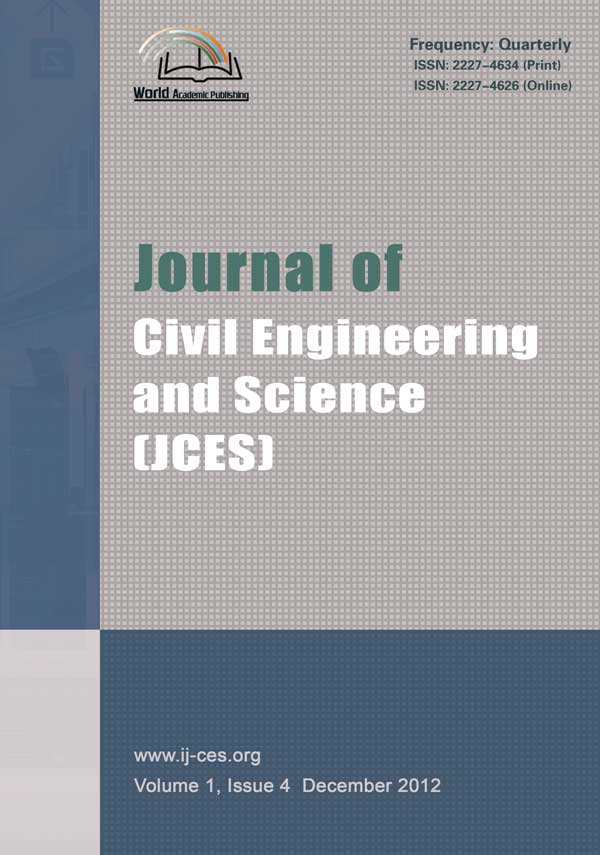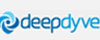Journal of Civil Engineering and ScienceJournal of Civil Engineering and Science(JCES) ISSN:2227-4634 (Print) ISSN:2227-4626(Online) Website: www.academicpub.org/jces/
 Turbulent Pipe-flow Computation Using the Rough Model Method (RMM) Full Paper(PDF, 692KB)Abstract: A new approach is presented to solve common straight pipe-flow problems, namely, computation of the discharge Q, computation of the internal diameter D and computation of the energy slope J. The theoretical approach is based on a referential rough pipe model characterized by an arbitrarily assigned relative roughness value, taken in the fully turbulence flow regime. Thus, the friction factor of Colebrook-White remains constant whatever the Reynolds number value. Hence, applying the Darcy-Weisbach formula, all parameters of the flow in the chosen model, such as the flowing discharge , the internal diameter and the energy slope , are then well defined. These allow a direct determination of the required value of Q, D and J by the use of a non-dimensional correction factors. The efficiency of the proposed approach is put forward through a practical application. Keywords:Rough Model Method; Turbulent Flow; Pipe; Friction Factor; Discharge; Energy Slope Author: Bachir ACHOUR1, Ali BEDJAOUI1 1.Research Laboratory in Subterranean and Surface Hydraulics (LARHYSS), University of Biskra References: P. K. Swamee, and A. K. Jain, “Explicit equations for pipe-flow problems,” J. Hydraulic Engineering, ASCE, vol. 102, HY5, pp.657-664, HY11, pp.1707-1709, 1976. P. K. Swamee and A. K. Jain, “Explicit equations for pipe-flow problems,” J. Hydraulic Engineering, ASCE, vol. 103, HY4, pp.460-463, 1977. P. K. Swamee and A. K. Jain, “Explicit equations for pipe-flow problems,” J. Hydraulic Engineering, ASCE, vol. 104 HY2, pp.300, 1978. W. H. Hager, “Die Berechnung turbulenter Rohrströmungen,” 3R-International, vol. 26, pp.116-121, 1987. R. O. Sinniger and W. H Hager, Constructions hydrauliques, 1ère Ed., Ed. Lausanne, Suisse: Presses Polytechniques Romandes, 1989. L. F. Moody, “Friction factors for pipe flow,” Transactions ASME, 1944. B. Achour and A. Bedjaoui, “Discussion of Explicit Solutions for Normal Depth Problem, by Prabhata K. Swamee, Pushpa N. Rathie,” J. Hydraulic Research, IAHR, vol. 44, pp.715-717, 2006.